# Hemisphere Shape

Here we will learn about the hemisphere shape, including what a hemisphere is and how to calculate the volume of a hemisphere and the surface area of a hemisphere.

There are also hemisphere shape worksheets based on Edexcel, AQA and OCR exam questions, along with further guidance on where to go next if you’re still stuck.

## What is a hemisphere shape?

A hemisphere shape is a three-dimensional shape that is half of a sphere.

To visualise this let’s look at a sphere. A sphere is a 3D shape where every point of its surface is equidistant (the same distance) from the centre of the sphere.

A hemisphere is half of a sphere. The prefix “hemi” means half.

You may have come across the word hemisphere in the real life context of planet Earth. We can split the world into 2 equal halves – the northern hemisphere and the southern hemisphere.

### What is a hemisphere shape?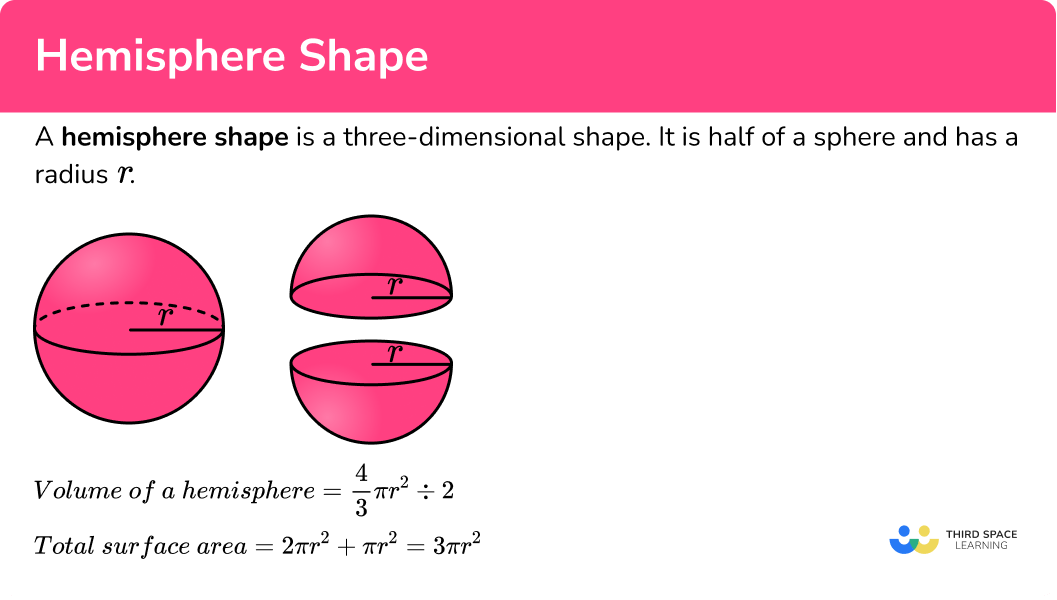### Properties of a hemisphere

A hemisphere is a geometric shape which has a curved surface area and a flat face, which is a circle.

A hemisphere has no vertices and one edge. It is not made up of polygons, so it is not a polyhedron.

Faces: 2

Edges: 1

Vertices: 0

### Volume of a hemisphere

The volume of a hemisphere is based on the volume of a sphere.

To calculate the volume of a sphere, where r is the radius of the sphere, we use the formula

\text{Volume of a sphere}=\frac{4}{3} \pi r^3.

To find the volume of a hemisphere we halve the volume of a sphere.

Here is the volume of a hemisphere formula, for a hemisphere with radius r,

\text{Volume of a hemisphere}=\frac{4}{3} \pi r^3\div 2

or

\text{Volume of a hemisphere}=\frac{2}{3} \pi r^{3}

Step-by-step guide: Volume of a hemisphere

### Surface area of a hemisphere

The surface area of a hemisphere is based on the surface area of a sphere.

To calculate the surface area of a sphere with radius r, we can use the formula

\text{Surface area of a sphere}=4\pi{r}^{2}.

So to find the curved surface area of the hemisphere we need to halve the surface area of the sphere.

4\pi{r}^{2}\div 2=2\pi{r}^{2}

However this would only give the curved surface area. If we want the total surface area, we need to add the area of the base.

The area of the base, which is a circle, is given by \pi r^{2}.

Here is the surface area of a hemisphere formula, for a hemisphere with radius r

\text{Total surface area of a hemisphere}=2\pi{r}^{2}+\pi{r}^{2}=3\pi{r}^{2} .

Step-by-step guide: Surface area of a hemisphere

## How to work out the volume or surface area of a hemisphere

In order to work out the volume or surface area of a hemisphere:

1. Write down the formula for the sphere.
2. Adapt the formula for the question.
3. Substitute in the value.

### Explain how to work out the volume or surface area of a hemisphere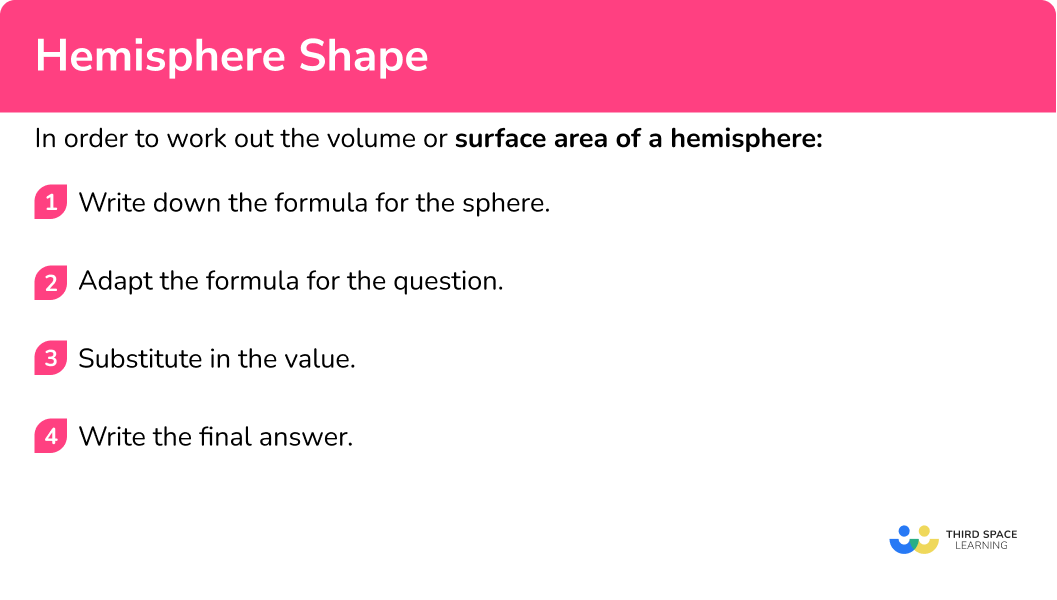## Hemisphere shape examples

### Example 1: volume

Find the volume of a hemisphere with radius 12.4 \ cm. Give your answer correct to 3 significant figures.

1. Write down the formula for the sphere.

We need to consider the formula for the volume of a sphere.

V=\frac{4}{3} \pi r^3

2Adapt the formula for the question.

The formula needs to be halved for the volume of a hemisphere.

V=\frac{4}{3} \pi r^3 \div 2

3Substitute in the value.

Then we substitute in the value 12.4 in place of r.

V=\frac{4}{3} \pi (12.4)^3 \div 2

This rounds to give 3990 \ cm^3 (to 3 significant figures) as the volume of the hemisphere.

### Example 2: volume in terms of pi

Find the volume of a hemisphere with radius 15 \ cm. Leave your answer in terms of \pi.

We need to consider the formula for the volume of a sphere.

V=\frac{4}{3} \pi r^3

The formula needs to be halved for the volume of a hemisphere.

V=\frac{4}{3} \pi r^3 \div 2

Then we substitute in the value 15 in place of r.

V=\frac{4}{3} \pi (15)^3 \div 2

The volume of the hemisphere is 2250\pi \ \mathrm{cm}^{3} .

### Example 3: curved surface area

Find the curved surface area of a hemisphere with diameter 4.5 \ m. Give your answer correct to 1 decimal place.

We need to consider the formula for the surface area of a sphere.

\text{Surface Area}=4\pi{r}^{2}

The formula needs to be halved for the curved surface area of a hemisphere.

\text{Curved Surface Area}=4\pi{r}^{2}\div 2=2\pi{r}^{2}

We have been given a diameter 4.5. We need to divide the diameter by 2 to get the radius. So we substitute in the value 2.25 in place of r.

\text{Curved Surface Area}=2\pi(2.25)^{2}

This rounds to give 31.8 \ m^2 (to 1 decimal place) as the curved surface area of the hemisphere.

### Example 4: total surface area

Find the total surface area of a hemisphere with radius 7.6 \ cm. Give your answer correct to 3 significant figures.

We need to consider the formula for the surface area of a sphere.

\text{Surface Area}=4\pi{r}^{2}

The formula needs to be halved for the curved surface area of a hemisphere.

\text{Curved Surface Area}=4\pi{r}^{2}\div 2=2\pi{r}^{2}

But, we need to also add the area of the circular base to get the total surface area of the hemisphere.

\text{Total Surface Area}=2\pi{r}^{2}+\pi{r}^{2}=3\pi{r}^{2}

Then we substitute in the value 7.6 in place of r.

\text{Total Surface Area}=3\pi(7.6)^{2}

This rounds to give 544 \ cm^2 (to 3 significant figures) as the total surface area of the hemisphere.

### Example 5: curved surface area in terms of pi

Find the curved surface area of a hemisphere with radius 8 \ cm. Leave your answer in terms of \pi.

We need to consider the formula for the surface area of a sphere.

\text{Surface Area}=4\pi{r}^{2}

The formula needs to be halved for the curved surface area of a hemisphere.

\text{Curved Surface Area}=4\pi{r}^{2}\div 2=2\pi{r}^{2}

Then we substitute in the value 8 in place of r.

\text{Curved Surface Area}=2\pi(8)^{2}

The curved surface area of the hemisphere is 128\pi \ \mathrm{cm}^{2} .

### Example 6: total surface area in terms of pi

Find the total surface area of a hemisphere with diameter 10 \ cm. Leave your answer in terms of \pi.

We need to consider the formula for the surface area of a sphere.

\text{Surface Area}=4\pi{r}^{2}

The formula needs to be halved for the curved surface area of a hemisphere.

\text{Curved Surface Area}=4\pi{r}^{2}\div 2=2\pi{r}^{2}

But, we need to also add the area of the circular base to get the total surface area of the hemisphere.

\text{Total Surface Area}=2\pi{r}^{2}+\pi{r}^{2}=3\pi{r}^{2}

We have been given a diameter 10. We need to divide the diameter by 2 to get the radius. So we substitute in the value 5 in place of r.

\text{Total Surface Area}=3\pi(5)^{2}

The total surface area of the hemisphere is 75\pi \ \mathrm{cm}^{2} .

### Common misconceptions

• Using the correct formula

There are several formulas that can be used in geometry, so we need to match the correct formula to the correct context.

• Total surface area or curved surface area

Double check whether the question requires you to find the curved surface area of a hemisphere or the total surface area of a hemisphere.

• Rounding

It is important to not round the answer until the end of the calculation. This will mean your final answer is accurate. It is useful to keep your answer in terms of \pi until you round the answer at the very end of the question.

• Make sure you have the correct units

For area we use square units such as cm^{2}.

For volume we use cube units such as cm^{3}.

• Using the radius or the diameter

It is a common error to mix up radius and diameter. Remember the radius is half of the diameter.

### Practice hemisphere shape questions

1. Find the volume of a hemisphere with radius 11.3 \ cm. Give your answer correct to 3 significant figures.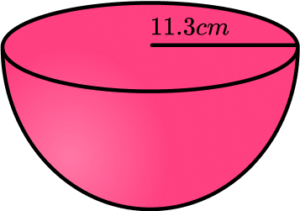3022 \ cm^33020 \ cm^36044 \ cm^36040 \ cm^3We need to halve the formula for the volume of a sphere, and then substitute the value of 11.3 in place of r.

\begin{aligned} V&=\frac{4}{3} \pi {r}^{3}\div 2\\\\ V&=\frac{4}{3} \times \pi \times (11.3)^{3}\div 2\\\\ V&=3021.996…\\\\ V&=3020 \ \mathrm{cm}^3 \ \text{(to 3 sf)} \end{aligned}

2. Find the volume of a hemisphere with diameter 4 \ cm. Leave your answer in terms of \pi.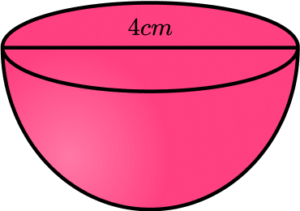\frac{16}{3}\pi \ \mathrm{cm}^3\frac{32}{3}\pi \ \mathrm{cm}^3\frac{256}{3}\pi \ \mathrm{cm}^3\frac{128}{3}\pi \ \mathrm{cm}^3We have been given the diameter 4, which we need to divide by 2 to get the radius. We need to halve the formula for the volume of a sphere, and then substitute the value of 2 in place of r.

\begin{aligned} V&=\frac{4}{3} \pi {r}^{3}\div 2\\\\ V&=\frac{4}{3} \times \pi \times (2)^{3}\div 2\\\\ V&=\frac{16}{3}\pi\\\\ V&=\frac{16}{3}\pi \ \mathrm{cm}^3 \end{aligned}

3. Find the curved surface area of a hemisphere with radius 12.8 \ cm. Give your answer correct to 3 significant figures.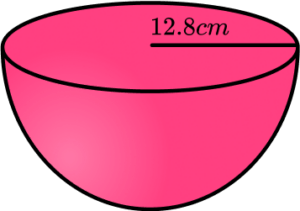515 \ cm^21030 \ cm^21540 \ cm^22060 \ cm^2We need to halve the formula for the surface area of a sphere to find the curved surface area of a hemisphere (CSA).

Then we substitute the value of 12.8 in place of r.

\begin{aligned} CSA&=4 \pi {r}^{2}\div 2\\\\ CSA&=2\pi {r}^{2}\\\\ CSA&=2\times \pi \times (12.8)^{2}\\\\ CSA&=1029.437…\\\\ CSA&=1030 \ \mathrm{cm}^2 \ \text{(to 3 sf)} \end{aligned}

4. Find the curved surface area of a hemisphere with radius 11 \ cm. Leave your answer in terms of \pi .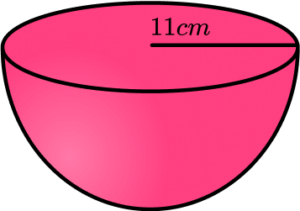242\pi \ \mathrm{cm}^2121\pi \ \mathrm{cm}^2968\pi \ \mathrm{cm}^244\pi \ \mathrm{cm}^2We need to halve the formula for the surface area of a sphere to find the curved surface area of a hemisphere (CSA).

Then we substitute the value of 11 in place of r.

\begin{aligned} CSA&=4 \pi {r}^{2}\div 2\\\\ CSA&=2\pi {r}^{2}\\\\ CSA&=2\times \pi \times (11)^{2}\\\\ CSA&=242\pi\\\\ CSA&=242\pi \ \mathrm{cm}^2 \end{aligned}

5. Find the total surface area of a hemisphere with radius 62 \ cm. Give your answer correct to 3 significant figures.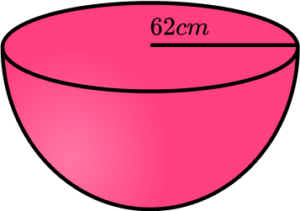48400 \ \mathrm{cm}^248300 \ \mathrm{cm}^236200 \ \mathrm{cm}^236300 \ \mathrm{cm}^2We need to halve the formula for the surface area of a sphere and add on the circular base to find the total surface area of a hemisphere (TSA).

Then we substitute the value of 62 in place of r.

\begin{aligned} TSA&=4 \pi {r}^{2}\div 2 + \pi {r}^{2}\\\\ TSA&=3\pi {r}^{2}\\\\ TSA&=3\times \pi \times (62)^{2}\\\\ TSA&=36228.8…\\\\ TSA&=36300 \ \mathrm{cm}^2 \ \text{(to 3 sf)} \end{aligned}

6. Find the total surface area of a hemisphere with radius 15 \ cm. Leave your answer in terms of \pi .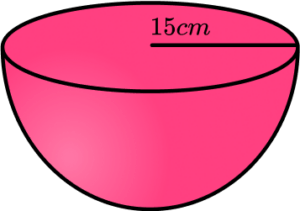225\pi \ \mathrm{cm}^2450\pi \ \mathrm{cm}^2675\pi \ \mathrm{cm}^2900\pi \ \mathrm{cm}^2We need to halve the formula for the surface area of a sphere and add on the circular base to find the total surface area of a hemisphere (TSA).

Then we substitute the value of 15 in place of r.

\begin{aligned} TSA&=4 \pi {r}^{2}\div 2 + \pi {r}^{2}\\\\ TSA&=3\pi {r}^{2}\\\\ TSA&=3\times \pi \times (15)^{2}\\\\ TSA&=675\pi\\\\ TSA&=675\pi \ \mathrm{cm}^2 \ \end{aligned}

### Hemisphere shape GCSE questions

1. Calculate the total surface area of a hemisphere with radius 5.2 \ cm.

(3 marks)

CSA: 196.897…cm^{2}, Circular base: 84.948… or 3\pi r^2

(1)

Evidence of adding CSA and circular area.

(1)

255 \ cm^2

(1)

2. Mary has a large hemisphere container in her garden.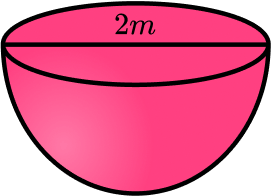It has a diameter of 2 metres.

She wants to fill the container with sand.

Sand comes in bags of 6000 \ cm^3 and the cost of a bag of sand is £2.95.

Calculate the total cost of the sand to fill the container.

(5 marks)

Radius is 100 \ cm .

(1)

V=\frac{4}{3}\times \pi \times {100}^{3} \div 2= 2 \ 094 \ 395.10…

(1)

2 \ 094 \ 395.10…\div 6000=349.06…

(1)

350\times \pounds2.95

(1)

£1032.50

(1)

3. A hemisphere has a total surface area of 1500 \ cm^2.

Calculate its diameter.

(4 marks)

1500=3\pi{r}^{2}

(1)

r=\sqrt{\frac{1500}{3\pi}}

(1)

12.6156…

(1)

25.231… = 25.2 \ cm

(1)

## Learning checklist

You have now learned how to:

• Calculate the volume of a hemisphere
• Calculate the curved surface area of a hemisphere
• Calculate the total surface area of a hemisphere

## Still stuck?

Prepare your KS4 students for maths GCSEs success with Third Space Learning. Weekly online one to one GCSE maths revision lessons delivered by expert maths tutors.

Find out more about our GCSE maths tuition programme.